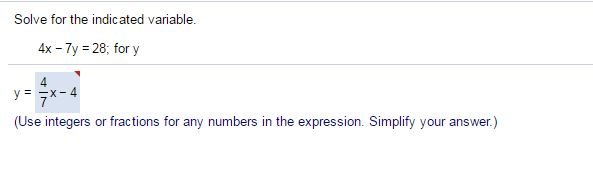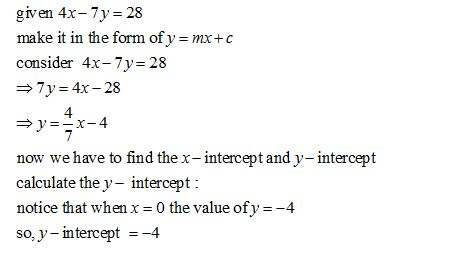Linear algebra

These questions are from millions of online tutorials and YouTube websites. I don’t know if I am allowed to ask experts to explain those questions in a different way. They are not homework assignments. I am trying to develop my own web site. Math’s has many ways to solve the problems. Just want to understand them.

1. My question is why the answer is not -4Who is Participating?
I wear a lot of hats...

"The solutions and answers provided on Experts Exchange have been extremely helpful to me over the last few years. I wear a lot of hats - Developer, Database Administrator, Help Desk, etc., so I know a lot of things but not a lot about one thing. Experts Exchange gives me answers from people who do know a lot about one thing, in a easy to use platform." -Todd S.

Commented:
As this equation has two variables, any solution of one variable will be another formula with factors rearranged...

The standard "line" formula is "y = mx + b"
This is called the "slope-intercept" form and expresses the value y in terms of the "slope"  (m in that equation)
and the "y intercept" (b in that equation)...

The y intercept is calculated by setting x to 0 as shown...

You can draw this standard graph by plotting the two intercept points:
x intercept:  (7,0)
y intercept:  (0, -4)
and drawing a straight line connecting them...

Not sure what additional explanations you need?

Experts Exchange Solution brought to you by